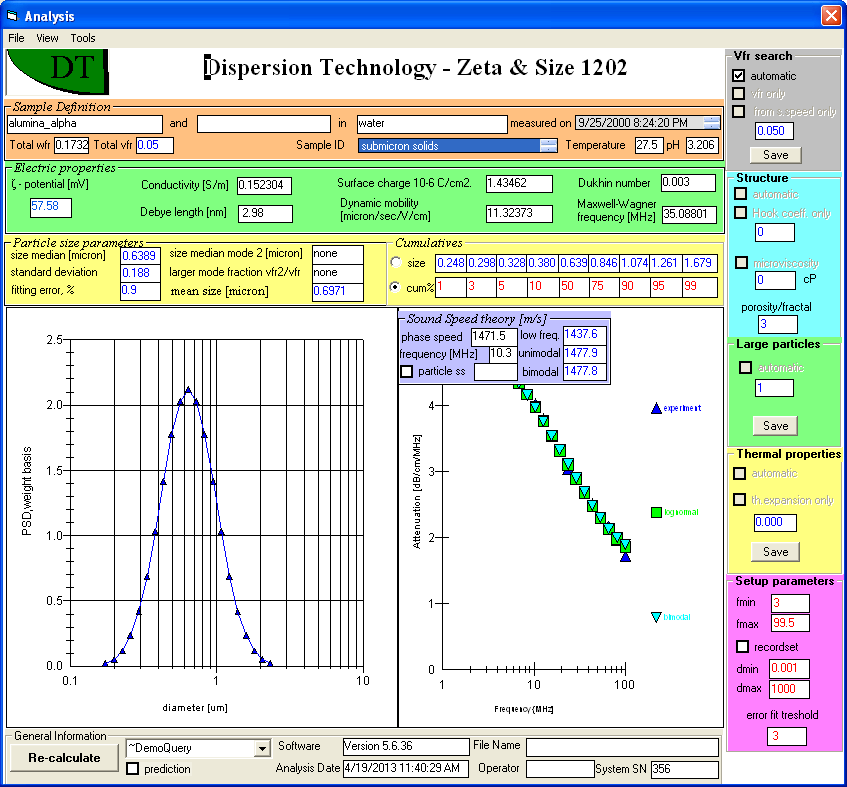# SOLIDS CONTENT

There are several methods for determining content of the dispersed phase in concentrated systems using our sensors.

1. Acoustic attenuation measurement allows extracting volume fraction together with particle size when size is within range from 0.1 to 1 micrometers. This method does not work beyond this size range due to problem of multiple solutions for the smooth attenuation curve.

2. Acoustic attenuation measurement allows determination of volume fraction when size is KNOWN even at single frequency measurement, which can be done in under a minute.

3. Sound speed measurement can be used for determining volume fraction of solids in some cases. There are ranges of solids content where sound speed is almost independent on volume fraction. This method does not work in such ranges.

4. Conductivity measurement can be used for determining volume fraction of solids, as described in the sections dedicated to Porosity measurements. The “Formation Factor“ of a dispersion or emulsion can be used for calculating volume fraction of solids. This method would need modifications for conducting particles, to account for the potential contribution of surface conductivity.

This Figure presents Report page of DTI software with tool box of VFR search on the right hand side top corner, which activates method 1. You can see that volume fraction calculated using this method (0.050) is identical to known volume fraction (0.05). This was verification test for the method 1.